# Poincaré space

Jump to: navigation, search

A Poincaré space of formal dimensionis a topological spacein which is given an elementsuch that the homomorphismgiven byis an isomorphism for each(here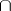is Whitney's product operation, the cap product). Moreover,is called the Poincaré duality isomorphism and the elementgenerates the group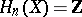. Any closed orientable-dimensional connected topological manifold is a Poincaré space of formal dimension;is taken to be an orientation (the fundamental class) of the manifold.

Letbe a finite cellular space imbedded in a Euclidean space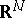of large dimension, letbe a closed regular neighbourhood of this imbedding and letbe its boundary. The standard mappingturns out to be a (Serre) fibration.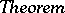:is a Poincaré space of formal dimensionif and only if the fibre of this fibration is homotopy equivalent to the sphere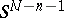. The described fibration which arises whenis a Poincaré space (the fibre of which is a sphere) is unique up to the standard equivalence and is called the normal spherical fibration, or the Spivak fibration, of the Poincaré space. Moreover, the cone of the projectionis the Thom space of the normal spherical fibration over.

If one restricts just to homology with coefficients in a certain field, then a so-called Poincaré space overis obtained.

One also considers Poincaré pairs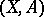(generalizations of the concept of a manifold with boundary), where for a certain generator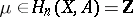and anythere is a Poincaré duality isomorphism: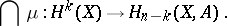Poincaré spaces naturally arise in problems on the existence and the classification of structures on manifolds. The problem of smoothing (triangulation) of a Poincaré space is also interesting, that is, to find a smooth (piecewise-linear), closed manifold that is homotopy equivalent to a given Poincaré space.

Sometimes, by-dimensional Poincaré space one means a closed-dimensional manifoldwith homology groups (cf. Homology group)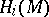isomorphic to the homology groupsof the-dimensional sphere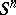; these are also called homology spheres.

A simply-connected Poincaré space is homotopy equivalent to a sphere. For a groupthat is realizable as the fundamental group of a certain Poincaré space one has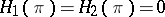, where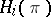are the homology groups of the group. Conversely, for any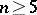and any finitely-presented groupwiththere exist an-dimensional Poincaré spacewith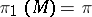.

For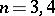these conditions are insufficient to realize the groupin the form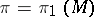. So, for example, the fundamental group of any three-dimensional Poincaré space admits a presentation with the same number of generators and relations. The only finite group which is realizable as the fundamental group of a three-dimensional Poincaré space is the binary icosahedral group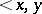: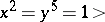, which is the fundamental group of the dodecahedral space — historically the first example of a Poincaré space.